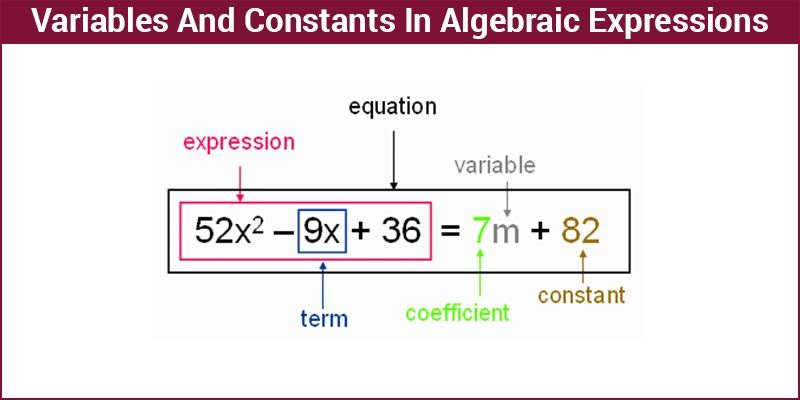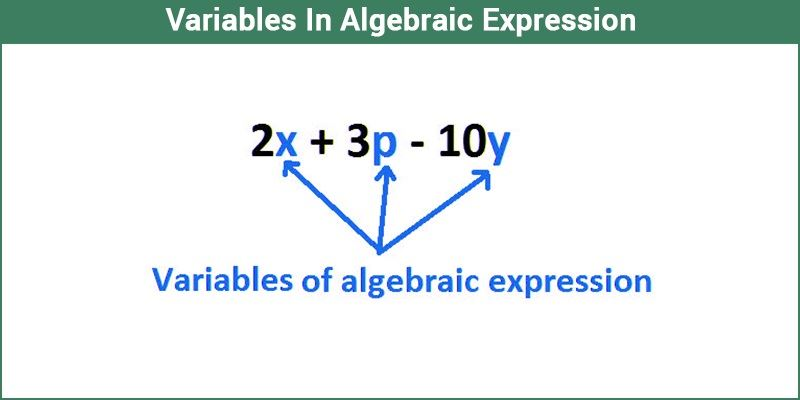# Variables And Constants In Algebraic Expressions

In Mathematics, an Algebra is a branch that deals with symbols, variables, numbers and the rules for manipulating it. It states the mathematical relationship are used to find the unknown value by creating expressions and equations. Now, consider the following algebraic expression,

2x3 + 3y2 + 4xy + 1

What are ‘x’ and ‘y’ over here? What are 1, 2, 3 and 4? They are letters and numbers, but what do they represent? To understand what they represent, you should first understand what variables and constants are. In this article, we will discuss what variables are, what are constants in Maths, expressions in detail.## What is Constant in Maths?

A constant is a value or number that never changes in expression; it’s constantly the same. For example, in the figure given above 36 and 82 are constant because its face value is 36 and 82 respectively. Its value never changes.

## What are Variables?Then what are ‘x’ and ‘y’? They are varying quantities because you can substitute different values for these and therefore they are called variable. A variable is a letter representing some unknown; a variable always represents a number, but it carries varying values when written in an expression. By convention, mathematicians usually assign letters(not mandatory) at the end of the alphabet (such as x, y, and z) to be variables.

All algebraic expressions and terms consist of at least one variable. It is the variable which distinguishes an algebraic expression from an arithmetic one. The presence of a variable in a mathematical expression enables infinite possibilities to determine the value of the expression.

### Algebraic Expressions and Terms

And what are algebraic expressions and terms? Is there a difference between the two? Let me give you an example. Let’s take an example of 2n and r².

Here 2n, r² and other examples like 4xy, 2x² and such are different terms. A term is a grouping together of one or more factors. Here multiplication is the only thing connecting the number with the variables.

Let’s take the number 4xy. Here 4, x and y are the factors of this number, where 4 is the coefficient or numerical factor. Didn’t understand? Okay, let me assign values. Let x = 2 and y = 3. Now, what is 4xy?

4 x 2 x 3 = ? Which is equal to 24. In this case, 4xy is 24 and 4, 2 and 3 are its factors. 4 again can be written as 2 x 2.

4xy takes different values with different values of x and y. So, the term is a combination of number and variable separated by multiplication or division. An expression is a combination of such terms.